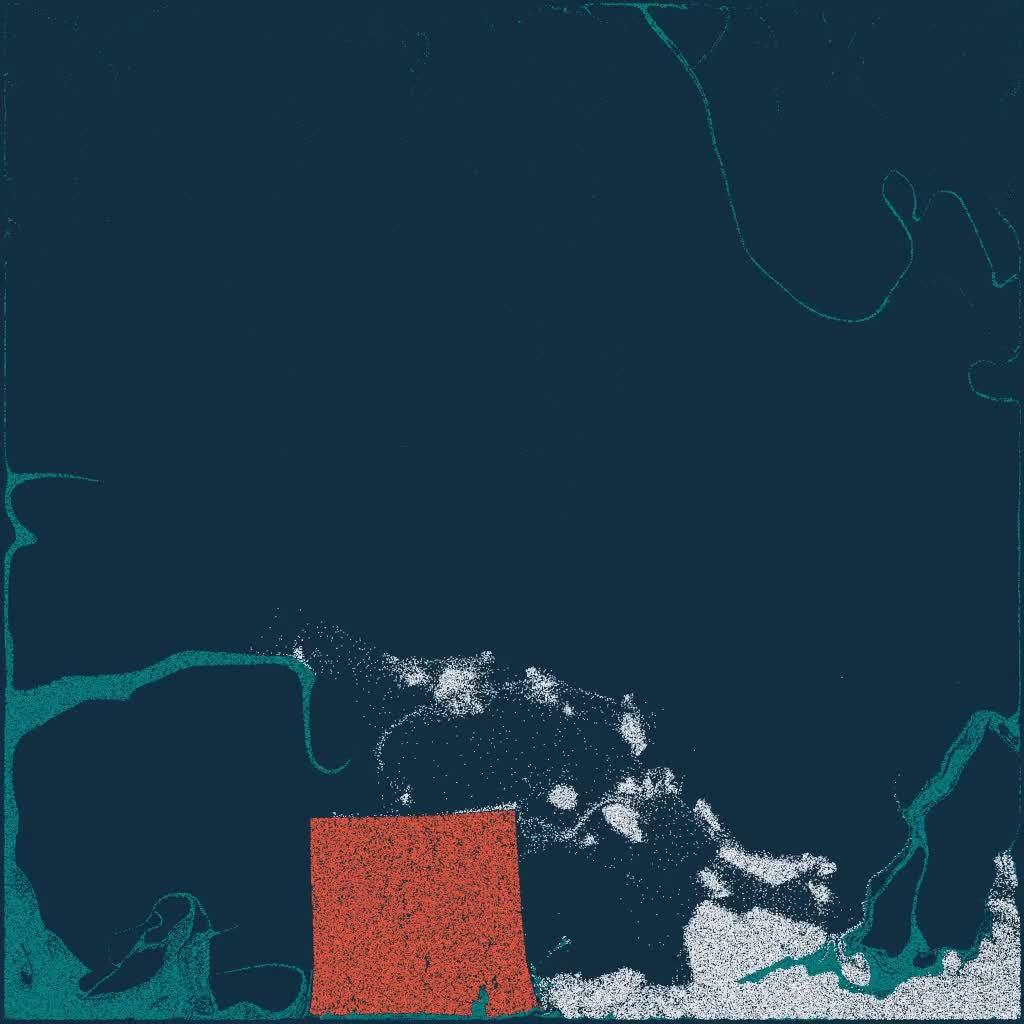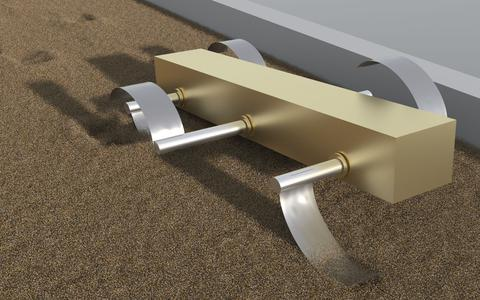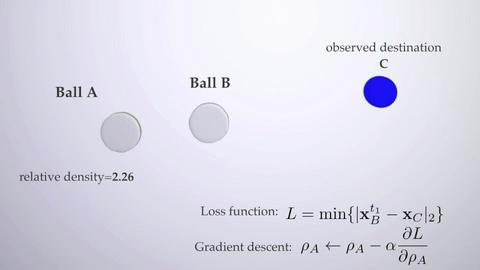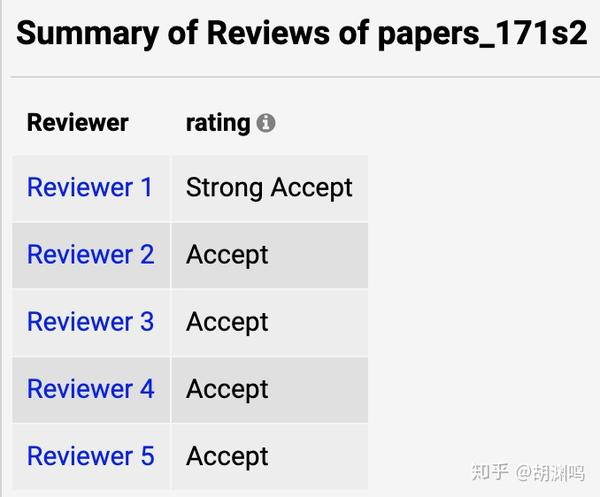## 目录

1. Material Point Method（物质点法）
2. Moving Least Squares Material Point Method（移动最小二乘物质点法）
3. Differentiable MLS-MPM (ChainQueen可微物理引擎)
4. “硬核”的计算机图形学
5. Taichi编程语言
6. Differentiable Programming (可微编程) 与 Taichi
7. import taichi as ti：假装成Python的Taichi语言
8. 总结

## 序14万个水，果冻，和雪“粒子”https://www.zhihu.com/video/1195682534812659712

import taichi as ti
import numpy as np
ti.init(arch=ti.gpu) # Try to run on GPU
quality = 1 # Use a larger value for higher-res simulations
n_particles, n_grid = 9000 * quality ** 2, 128 * quality
dx, inv_dx = 1 / n_grid, float(n_grid)
dt = 1e-4 / quality
p_vol, p_rho = (dx * 0.5)**2, 1
p_mass = p_vol * p_rho
E, nu = 0.1e4, 0.2 # Young's modulus and Poisson's ratio
mu_0, lambda_0 = E / (2 * (1 + nu)), E * nu / ((1+nu) * (1 - 2 * nu)) # Lame parameters
x = ti.Vector(2, dt=ti.f32, shape=n_particles) # position
v = ti.Vector(2, dt=ti.f32, shape=n_particles) # velocity
C = ti.Matrix(2, 2, dt=ti.f32, shape=n_particles) # affine velocity field
F = ti.Matrix(2, 2, dt=ti.f32, shape=n_particles) # deformation gradient
material = ti.var(dt=ti.i32, shape=n_particles) # material id
Jp = ti.var(dt=ti.f32, shape=n_particles) # plastic deformation
grid_v = ti.Vector(2, dt=ti.f32, shape=(n_grid, n_grid)) # grid node momentum/velocity
grid_m = ti.var(dt=ti.f32, shape=(n_grid, n_grid)) # grid node mass

@ti.kernel
def substep():
for i, j in grid_m:
grid_v[i, j] = [0, 0]
grid_m[i, j] = 0
for p in x: # Particle state update and scatter to grid (P2G)
base = (x[p] * inv_dx - 0.5).cast(int)
fx = x[p] * inv_dx - base.cast(float)
# Quadratic kernels  [http://mpm.graphics   Eqn. 123, with x=fx, fx-1,fx-2]
w = [0.5 * ti.sqr(1.5 - fx), 0.75 - ti.sqr(fx - 1), 0.5 * ti.sqr(fx - 0.5)]
F[p] = (ti.Matrix.identity(ti.f32, 2) + dt * C[p]) @ F[p] # deformation gradient update
h = ti.exp(10 * (1.0 - Jp[p])) # Hardening coefficient: snow gets harder when compressed
if material[p] == 1: # jelly, make it softer
h = 0.3
mu, la = mu_0 * h, lambda_0 * h
if material[p] == 0: # liquid
mu = 0.0
U, sig, V = ti.svd(F[p])
J = 1.0
for d in ti.static(range(2)):
new_sig = sig[d, d]
if material[p] == 2:  # Snow
new_sig = min(max(sig[d, d], 1 - 2.5e-2), 1 + 4.5e-3)  # Plasticity
Jp[p] *= sig[d, d] / new_sig
sig[d, d] = new_sig
J *= new_sig
if material[p] == 0:  # Reset deformation gradient to avoid numerical instability
F[p] = ti.Matrix.identity(ti.f32, 2) * ti.sqrt(J)
elif material[p] == 2:
F[p] = U @ sig @ V.T() # Reconstruct elastic deformation gradient after plasticity
stress = 2 * mu * (F[p] - U @ V.T()) @ F[p].T() + ti.Matrix.identity(ti.f32, 2) * la * J * (J - 1)
stress = (-dt * p_vol * 4 * inv_dx * inv_dx) * stress
affine = stress + p_mass * C[p]
for i, j in ti.static(ti.ndrange(3, 3)): # Loop over 3x3 grid node neighborhood
offset = ti.Vector([i, j])
dpos = (offset.cast(float) - fx) * dx
weight = w[i] * w[j]
grid_v[base + offset] += weight * (p_mass * v[p] + affine @ dpos)
grid_m[base + offset] += weight * p_mass
for i, j in grid_m:
if grid_m[i, j] > 0: # No need for epsilon here
grid_v[i, j] = (1 / grid_m[i, j]) * grid_v[i, j] # Momentum to velocity
grid_v[i, j] -= dt * 50 # gravity
if i < 3 and grid_v[i, j] < 0:          grid_v[i, j] = 0 # Boundary conditions
if i > n_grid - 3 and grid_v[i, j] > 0: grid_v[i, j] = 0
if j < 3 and grid_v[i, j] < 0:          grid_v[i, j] = 0
if j > n_grid - 3 and grid_v[i, j] > 0: grid_v[i, j] = 0
for p in x: # grid to particle (G2P)
base = (x[p] * inv_dx - 0.5).cast(int)
fx = x[p] * inv_dx - base.cast(float)
w = [0.5 * ti.sqr(1.5 - fx), 0.75 - ti.sqr(fx - 1.0), 0.5 * ti.sqr(fx - 0.5)]
new_v = ti.Vector.zero(ti.f32, 2)
new_C = ti.Matrix.zero(ti.f32, 2, 2)
for i, j in ti.static(ti.ndrange(3, 3)): # loop over 3x3 grid node neighborhood
dpos = ti.Vector([i, j]).cast(float) - fx
g_v = grid_v[base + ti.Vector([i, j])]
weight = w[i] * w[j]
new_v += weight * g_v
new_C += 4 * inv_dx * weight * ti.outer_product(g_v, dpos)
v[p], C[p] = new_v, new_C
x[p] += dt * v[p] # advection

group_size = n_particles // 3
@ti.kernel
def initialize():
for i in range(n_particles):
x[i] = [ti.random() * 0.2 + 0.3 + 0.10 * (i // group_size), ti.random() * 0.2 + 0.05 + 0.32 * (i // group_size)]
material[i] = i // group_size # 0: fluid 1: jelly 2: snow
v[i] = ti.Matrix([0, 0])
F[i] = ti.Matrix([[1, 0], [0, 1]])
Jp[i] = 1
initialize()
gui = ti.GUI("Taichi MLS-MPM-99", res=512, background_color=0x112F41)
for frame in range(20000):
for s in range(int(2e-3 // dt)):
substep()
colors = np.array([0x068587, 0xED553B, 0xEEEEF0], dtype=np.uint32)
gui.show() # Change to gui.show(f'{frame:06d}.png') to write images to disk

# 支持Linux, OS X and Windows
python3 -m pip install taichi

（注：taichi最新版本为v0.6.6。部分网友反应国内PyPI镜像存在一定程度版本滞后现象。最新代码链接：纯流体88行版多材料99行版可交互128行版

## Material Point Method（物质点法）

Material Point Method (MPM）是一种模拟连续介质的方法，最早被Sulsky等人在1995年发明[Application of a particle-in-cell method to solid mechanics]。由于在计算中同时使用了Lagrangian particle和Eulerian grid，他被归类到Hybrid Lagrangian-Eulerian Simulation Method。这类方法最早可以追溯到Particle-in-Cell (PIC, The particle-in-cell method for numerical solution of problems in fluid dynamics, 1963) 和Fluid Implicit Particles (FLIP, A method for adaptively zoned, particle-in-cell calculations of fluid flows in two dimensions，1986)。熟悉有限元的同学可以把MPM里面的grid nodes对应到FEM里面的DOFs, 把MPM里面的particles对应到FEM里面的quadrature points。和FEM里使用显式网格测策略不同，作为一种Element-Free Galerkin（EFG）法，MPM里面并没有显式的Elements和Lagrangian grid，只有能够随意移动的粒子作为quadrature points。这样的特性使得它非常适合处理大形变，而其背景网格带来的自动碰撞处理、多材料耦合。由于离散化出自weak form，这使得MPM的physical accuracy有了保证。

## Moving Least Squares Material Point Method（移动最小二乘物质点法）

2017年大四暑假，我在宾夕法尼亚大学visit蒋老师。我们设计了一个新算法，Moving Least Squares MPM (MLS-MPM)。MLS-MPM不仅性能比之前的state of the art高两倍，而且代码短了很多，非常容易实现。为了加速我们的实验，让MPM跑的快一点，我花了点时间手写SIMD intrinsics，把它加速了6倍。在这个基础上，MLS-MPM在算法上的改进还能进一步提高两倍的性能。

[SIGGRAPH 2018] 移动最小二乘物质点法与兼容性粒子网格法（双向流体/固体耦合）_哔哩哔哩 (゜-゜)つロ 干杯~-bilibiliwww.bilibili.com## Differentiable MLS-MPM (ChainQueen可微物理引擎)

[ICRA 2019] ChainQueen（乾坤）可微软体物理引擎_哔哩哔哩 (゜-゜)つロ 干杯~-bilibiliwww.bilibili.com## “硬核”的计算机图形学“几百个.......通过”: 那年SIGGRAPH评分系统有6挡: strong rej, reject, weak rej, weak acc, accept, strong acc。这几年规则一直在变，不知道今年有几档...

Comments about Video: The video would be a great place to provide an overview and a big picture of the entire framework, and could also help the reader understand details that were not adequately explained in the paper. Unfortunately, this was not done, the video is extremely short, and only shows some examples.

[SIGGRAPH Asia 2019] Taichi编程语言：高性能稀疏视觉计算_哔哩哔哩 (゜-゜)つロ 干杯~-bilibiliwww.bilibili.com## Differentiable Programming (可微编程) 与 DiffTaichi[项目主页]

[ICLR 2020] DiffTaichi：基于Taichi的可微编程和可微物理引擎_哔哩哔哩 (゜-゜)つロ 干杯~-bilibiliwww.bilibili.com## import taichi as ti（Taichi与Python的无缝衔接）

DiffTaichi完成到现在，我一直在做一些语法、标准库（主要是linear algebra）方面的设计，编译器实现方面的工程工作。MIT内部有3个项目在使用Taichi开发，校外还有两三个组在试用Taichi。我现在每天的工作基本上就是为他们提供支持、开发新的feature方便这些用户。

1. 由于Python本身是一门很容易学、普及程度很高的语言，伪装成Python以后的Taichi语言很容易学习；
2. Python自带包管理系统，这使得作为编程语言的Taichi可以伪装成一个普通的Python package；
3. 已有的很多Python IDE可以直接拿来当做Taichi的IDE；
4. 这样设计以后很多常用的Python包，如numpy、matplotlib、PyTorch都可以和Taichi无缝衔接。

2. LLVM对SIMD的良好支持（大部分SIMD intrinsics不用手写了）
3. 基于LLVM开发的系统的高portability（ship一个包含了statically-compiled LLVM的shared object比要求用户装gcc/clang-7/8容易多了）。

1. 你用啥操作系统？
2. glibc版本是啥？“ld --version”输出给我看看？
3. CPU哪一年的？AVX2支持吗？
4. GPU啥型号？compute capability多少？OpenGL 4.1支持吗？
5. GLFW，GLEW装了吗？
6. gcc/clang版本对不？C++14支持吗？
7. ...

## 附录：

Taichi论文摘要：(SIGGRAPH Asia 2019) 三维体积数据通常具有空间稀疏性。为了利用这种性质，计算机图形学社区开发了层级体素稀疏数据结构，如SPGrid、VDB和八叉树等。但是，由于其内在复杂性和额外开销，开发、应用这些高性能数据结构有很多挑战。我们提出Taichi，一个新的面向（稀疏）数据的编程语言，大大降低了空间稀疏数据结构的开发、使用成本。由于Taichi实现了算法和数据结构的解耦，使用者可以快速尝试不同数据结构，以在特定问题和体系结构上找到最优数据结构。语言前端提供给用户易用的接口，使得用户可以以访问稠密数据结构的方式访问稀疏数据结构，大大提高了代码可读性和生产力。Taichi编译器使用对数据结构的语义和下标分析来优化程序的局部性，移除多余数据结构遍历，以及进行自动内存管理和并行化、向量化。在x86_64和CUDA体系结构上，只需要1/10的代码，Taichi程序就能比手动优化的稀疏计算基准程序快4.55倍，测试程序包括物质点法、有限元模拟、多重网格泊松方程求解，真实感渲染，和3D稀疏卷积神经网络等。项目主页和代码：github.com/taichi-dev/t

DiffTaichi论文摘要：(ICLR 2020) 基于Taichi，我们提出可微编程语言DiffTaichi，用于构建端到端可微程序。和目前常用的可微编程工具如TensorFlow、PyTorch相比，DiffTaichi更适合构建比常用操作（如卷积、BN等）更不规则的可微运算符，比如可微物理引擎中的粒子网格交互，网格采样等等。DiffTachi的自动微分系统使用“两个尺度”设计：底层通过源代码变换保持并行性和算术强度(arithmetic intensity)，上层通过一个轻量级的磁带(Tape)来记录大内核(Megakernel)的启动。由于省去了枯燥的手动求导过程，DiffTaichi程序比CUDA短4.2倍并具有相同的性能；同时由于其Megakernel的设计，在编写复杂可微程序时，DiffTaichi比TensorFlow快188倍、比PyTorch快13.4倍。我们用DiffTaichi实现了10个不同的可微物理引擎。将神经网络嵌入其中，控制器优化可以在几十个梯度下降迭代中完成，这比增强学习(RL)收敛速度快若干数量级。项目主页和代码：github.com/yuanming-hu/

(标题图来自互联网)